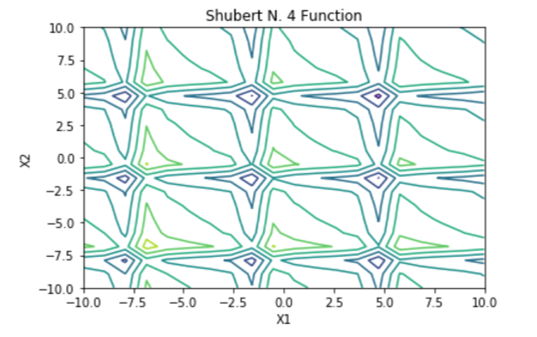top of page
Search

# Python Implementation of Shubert N. 4 Function

Updated: Jul 18, 2021Mathematical DefinitionInput Domain

The function can be defined on any input range but it is usually evaluated on xi ∈ [-10,10] for

i = 1,...,n.

Global Minima

The function has one global minimum f(x*) ≈−25.740858.

Characteristics

The Shubert function has several local and global minima.

The function is continuous.

The function is not convex.

The function is defined on n-dimensional space.

The function is multimodal.

The function is differentiable.

The function is separable.

Python Implementation

```% Please forward any comments or bug reports in chat
Copyright 2021. INDUSMIC PRIVATE LIMITED.THERE IS NO WARRANTY, EXPRESS OR IMPLIED. WE DO NOT ASSUME ANY LIABILITY FOR THE USE OF THIS PROGRAM. If software is modified to produce derivative works, such modified software should be clearly marked. Additionally, user can redistribute it and/or modify it under the terms of the GNU General Public License. This program is distributed in the hope that it will be useful, but WITHOUT ANY WARRANTY. See the GNU General Public License for more details.
% for any support connect with us on help.indusmic@gmail.com
% Author: SHIVANGI CHANDRA DUBEY

#For n=2
#shubertn4 accepts the   values of 2 MxM dimension matrices X1, X2
#it returns the   computation of the matrices in an MxM matrix Z
#the function is then   plotted using (X1,X2,Z)
#thus giving us a   contour plot

from mpl_toolkits import mplot3d
%matplotlib inline
import numpy as np
import matplotlib.pyplot as plt
from matplotlib import cm

def shubertn4(x1,x2):
y=0;
for j in range(1,6):
y=y+j*np.cos((j+1)*x1+j)+   j*np.cos((j+1)*x2+j)
return y

x1=np.linspace(-10,10,20)
x2=np.linspace(-10,10,20)

X1,X2=np.meshgrid(x1,x2)
Z=shubertn4(X1,X2)

def plotFunction(e,a):
fig=plt.figure(figsize = [12,8])
ax=plt.axes(projection='3d')
surf=ax.plot_surface(X1,X2,Z,cmap=cm.coolwarm)
ax.view_init(elev=e,azim=a)
ax.set_xlabel('X1')
ax.set_ylabel('X2')
ax.set_zlabel('fx')
ax.set_title('Shubert N. 4 Function')
fig.colorbar(surf, shrink=0.5, aspect=5)
plt.show()
plt.contour(X1, X2, Z)
plt.show()

from ipywidgets import interactive
iplot=interactive(plotFunction,
e=(-90,90,5),
a=(-90,90,5))
iplot

```

References:

 Survajonic, Sonja & Bingham, Derek, “Virtual Library of Simulation Experiments”, sfu.ca,

https://www.sfu.ca/~ssurjano/optimization.html